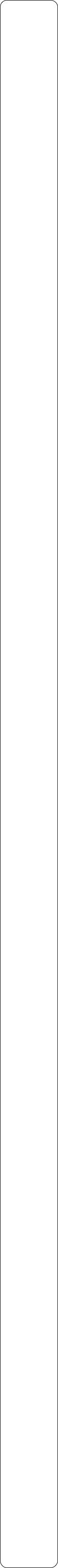Year 6 ObjectivesPlace ValuePre Tasks

(PitStops)

Main Tasks

Addition and SubtractionPre Tasks

(PitStops)

Main Tasks

Multiplication and Division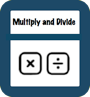Number - Fractions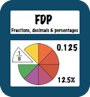Pre Tasks

(PitStops)

Main Tasks

Pre Tasks

(PitStops)

Main Tasks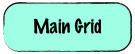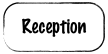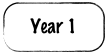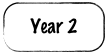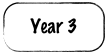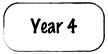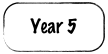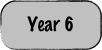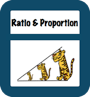Pre Tasks

(PitStops)

Main TasksAlgebra

Ratio and Proportion- Read, write, order and compare numbers up to 10 000 000 and determine the value of each digit

- Round any whole number to a required degree of accuracy

1. -Use negative numbers in context, and calculate intervals across zero

-  Solve number and practical problems that involve all of the above.- Multiply multi-digit numbers up to 4 digits by a two-digit whole number using the formal written method of long multiplication

- Divide numbers up to 4 digits by a two-digit whole number using the formal written method of long division, and interpret remainders as whole number remainders, fractions, or by rounding, as appropriate for the context

- Divide numbers up to 4 digits by a two-digit number using the formal written method of short division where appropriate, interpreting remainders according to the context

- Perform mental calculations, including with mixed operations and large numbers

- Identify common factors, common multiples and prime numbers

- Use their knowledge of the order of operations to carry out calculations involving the four operations

- Solve addition and subtraction multi-step problems in contexts, deciding which operations and methods to use and why

- Solve problems involving addition, subtraction, multiplication and division

- Use estimation to check answers to calculations and determine, in the context of a problem, an appropriate degree of accuracy- Use common factors to simplify fractions; use common multiples to express fractions in the same denomination

- Compare and order fractions, including fractions > <

- Add and subtract fractions with different denominators and mixed numbers, using the concept of equivalent fractions

- Multiply simple pairs of proper fractions, writing the answer in its simplest form [for example, ¼ x ½ = ⅛]

- Divide proper fractions by whole numbers [for example, ⅓ ÷ 2 = ⅙ ]

- Associate a fraction with division and calculate decimal fraction equivalents [for example, 0.375] for a simple fraction [for example, ⅜]

- Identify the value of each digit in numbers given to three decimal places and multiply and divide numbers by 10, 100 and 1000 giving answers up to three decimal places

- Multiply one-digit numbers with up to two decimal places by whole numbers

- Use written division methods in cases where the answer has up to two decimal places

- Solve problems which require answers to be rounded to specified degrees of accuracy

- Recall and use equivalences between simple fractions, decimals and percentages, including in different contexts- Solve problems involving the relative sizes of two quantities where missing values can be found by using integer multiplication and division facts

- Solve problems involving the calculation of percentages [for example, of measures, and such as 15% of 360] and the use of percentages for comparison

- Solve problems involving similar shapes where the scale factor is known or can be found

- Solve problems involving unequal sharing and grouping using knowledge of fractions and multiples- Use simple formulae

- Generate and describe linear number sequences

- Express missing number problems algebraically

- Find pairs of numbers that satisfy an equation with two unknowns

- Enumerate possibilities of combinations of two variables.Solutions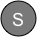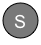StopsGap

StopsGap

StopsGap

StopsGap

StopsGap